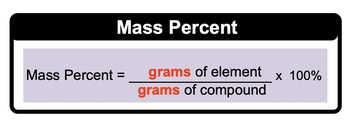## General Chemistry

Learn the toughest concepts covered in Chemistry with step-by-step video tutorials and practice problems by world-class tutors

3. Chemical Reactions

# Mass Percent

Mass Percent is the percentage of a particular element within a compound.

Mass Percent
1
concept

## Mass Percent Concept38s
Play a video:
here. We're going to say that mass percent, also known as weight percent, is the percentage of a given element in a compound. Now we're gonna stay here. It deals with the Moller Mass in grams of the selected element and one more of the compound. So this translate to say that mass percent equals the mass or grands of an element divided by the grams off the compound used and then multiplying that by 100. Using this helps us to determine the percent composition of any particular element within a given compound.2
example

## Mass Percent Example1m
Play a video: